Vector Calculus - 7

# Vector Calculus - 7

Test Description

## 20 Questions MCQ Test Topic-wise Tests & Solved Examples for IIT JAM Mathematics | Vector Calculus - 7

Vector Calculus - 7 for Mathematics 2023 is part of Topic-wise Tests & Solved Examples for IIT JAM Mathematics preparation. The Vector Calculus - 7 questions and answers have been prepared according to the Mathematics exam syllabus.The Vector Calculus - 7 MCQs are made for Mathematics 2023 Exam. Find important definitions, questions, notes, meanings, examples, exercises, MCQs and online tests for Vector Calculus - 7 below.
Solutions of Vector Calculus - 7 questions in English are available as part of our Topic-wise Tests & Solved Examples for IIT JAM Mathematics for Mathematics & Vector Calculus - 7 solutions in Hindi for Topic-wise Tests & Solved Examples for IIT JAM Mathematics course. Download more important topics, notes, lectures and mock test series for Mathematics Exam by signing up for free. Attempt Vector Calculus - 7 | 20 questions in 60 minutes | Mock test for Mathematics preparation | Free important questions MCQ to study Topic-wise Tests & Solved Examples for IIT JAM Mathematics for Mathematics Exam | Download free PDF with solutions
 1 Crore+ students have signed up on EduRev. Have you?
Vector Calculus - 7 - Question 1

### A vector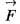is said to be irrotational vector, if

Vector Calculus - 7 - Question 2

### The plane containing principal, normal and binormal is called

Vector Calculus - 7 - Question 3

### Maximum value of directional derivative of f= x2yz at the point (1, 4,1) is

Vector Calculus - 7 - Question 4

If V is the volume enclosed by surface S and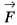=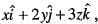then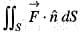is

Vector Calculus - 7 - Question 5

If C is a smooth curve in R3 from (–1, 0, 1) to (1, 1, –1), then the value of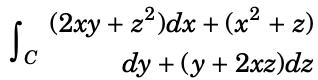is

Detailed Solution for Vector Calculus - 7 - Question 5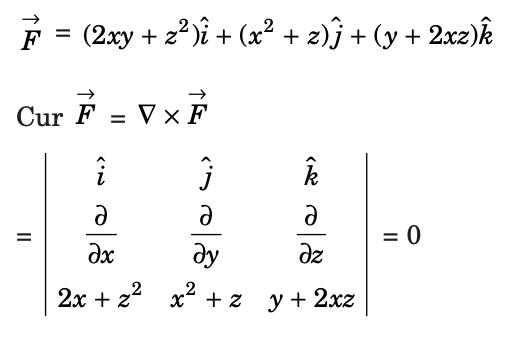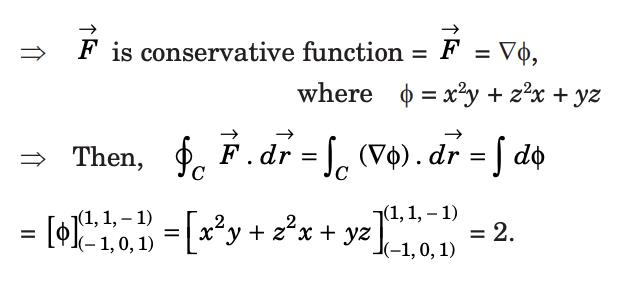Vector Calculus - 7 - Question 6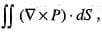where P is a vector, is equal to

Vector Calculus - 7 - Question 7

if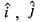and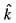be the set of orthonormal unit vectors, then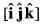is

Vector Calculus - 7 - Question 8

Stoke’s theorem is

Detailed Solution for Vector Calculus - 7 - Question 8

Explanation : The Stoke’s theorem states that “the surface integral of the curl of a function over a surface bounded by a closed surface is equal to the line integral of the particular vector function around that surface.”

Stokes theorem says that ∫F·dr = ∬curl(F)·n ds.

Vector Calculus - 7 - Question 9

The relation between the line integral and the surface integral is

Vector Calculus - 7 - Question 10

The divergence of the vector field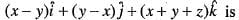Vector Calculus - 7 - Question 11

If Φ(x, y, z) = 3x2y - y3z then the value of grad Φ at the point (1,- 2 ,- 1 ) is

Vector Calculus - 7 - Question 12

If Φ is a differentiable scalar point function, then the value of curl grad Φ is

Vector Calculus - 7 - Question 13

Ifthen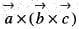is

Vector Calculus - 7 - Question 14

A particle moves along the curve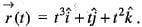Acceleration of the particle in the direction of the motion is

Vector Calculus - 7 - Question 15

Using stokes' theorem evaluate the line integral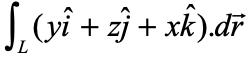, where L is the intersection of x2 + y2 + z2 = 1 and x + y = 0 traversed in the clockwise direction when viewed from the point (1, 1, 0)

Detailed Solution for Vector Calculus - 7 - Question 15

By stoke’s theorem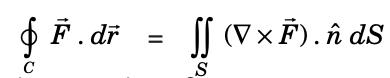where L is the intersection of x2 + y2 + z2 = 1 and x + y = 0.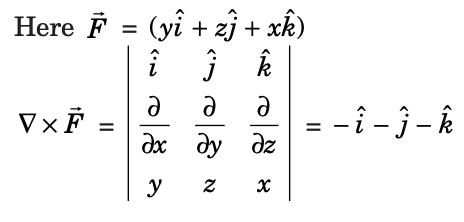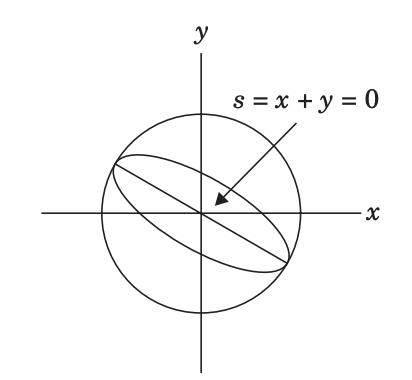The outward unit normal vector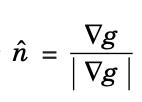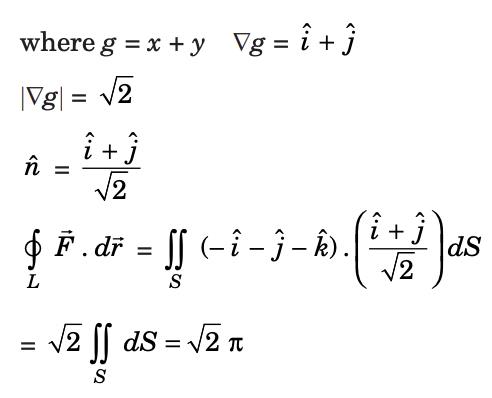Vector Calculus - 7 - Question 16

Gauss’s divergence theorem can be written a

Detailed Solution for Vector Calculus - 7 - Question 16

The divergence theorem states that the surface integral of the normal component of a vector point function “F” over a closed surface “S” is equal to the volume integral of the divergence of F⃗  taken over the volume “V” enclosed by the surface S. Thus, the divergence theorem is symbolically denoted as :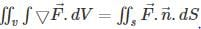Vector Calculus - 7 - Question 17

Let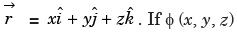is a solution of the Laplace equation then the vector field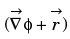is

Detailed Solution for Vector Calculus - 7 - Question 17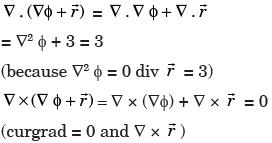⇒ Divergence is non-zero and curl is zero,
Hence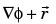is not solenoidal but irrotational.

Vector Calculus - 7 - Question 18

Let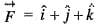be a vector field.
Q. The value of div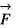is

Detailed Solution for Vector Calculus - 7 - Question 18

Given that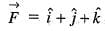Therefore,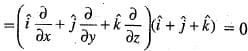Vector Calculus - 7 - Question 19

Letbe a vector field.
Q. The value of curl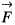is

Detailed Solution for Vector Calculus - 7 - Question 19

Given that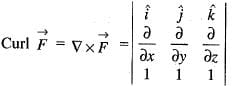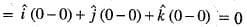Vector Calculus - 7 - Question 20

Let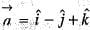and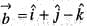Q. The value of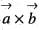is

Detailed Solution for Vector Calculus - 7 - Question 20

Given that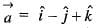and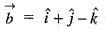Therefore,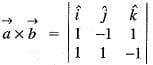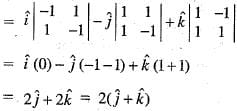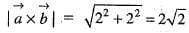## Topic-wise Tests & Solved Examples for IIT JAM Mathematics

27 docs|150 tests
 Use Code STAYHOME200 and get INR 200 additional OFF Use Coupon Code
Information about Vector Calculus - 7 Page
In this test you can find the Exam questions for Vector Calculus - 7 solved & explained in the simplest way possible. Besides giving Questions and answers for Vector Calculus - 7, EduRev gives you an ample number of Online tests for practice

## Topic-wise Tests & Solved Examples for IIT JAM Mathematics

27 docs|150 tests